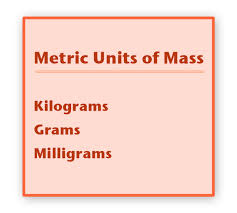## Preview

To start this introduction you need to know there are three types of metric units: Metric Units of Length,
Metric Unit of Capacity, and Metric Units of Mass. The first is units of length and that includes of:

Next is the units of capacity which includes of:Third is the units of mass which include of:All of these units are uses of everyday life so knowing them really gives you a benefit of calculating things more easily.
For example you will use meters from the units of lengths to find out how much you walked.
You will use cups from the units of capacity to calculate how much you need to put in your recipe.
You will use kilograms from the units of mass to find out how much weight you have lost.
Now try some of these and make sure to watch the video on YouTube.

Sheet 1 What are Metric Units